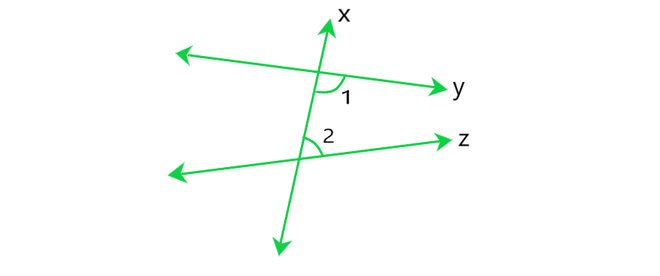Open In App
Related Articles
• NCERT Solutions for Class 9 Maths

# Class 9 NCERT Solutions- Chapter 5 Introduction to Euclid’s Geometry – Exercise 5.2

### Question 1: How would you rewrite Euclid’s fifth postulate so that it would be easier to understand?

Solution:

Euclid’s fifth postulate:

Euclid’s fifth postulate says that If a straight line falling on two straight lines makes the interior angles on the same side of it taken together less than two right angles, then the two straight lines, if the lines produced indefinitely, meet on that side on which the sum of angles is less than two right angles.

i.e., the Euclid’s fifth postulate tell us about parallel lines.

Parallel lines are the lines which do not intersect each other ever and are always set at a perpendicular distance apart from each other.

Parallel lines can be two or more lines.

A: If X does not lie on the line A then we can draw a line through X which will be parallel to that of the line A.

B: There can be only one line that can be drawn through the point X which is parallel to the line A.

Therefore, Two distinct intersecting lines cannot be parallel to the same line.

### Question 2: Does Euclid’s fifth postulate imply the existence of parallel lines? Explain.

Solution:Yes. Euclid’s fifth postulate imply the existence of the parallel lines.

According to Euclid’s fifth postulate when a line x falls on a line y and z such that ∠1+ ∠2< 180°.

Then, line y and line z on producing further will meet in the side of ∠1 arid ∠2 which is less than 180°.

Therefore, we find that the lines which are not according to Euclid’s fifth postulate. i.e., ∠1 + ∠2 = 180°, do not intersect.